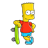Tuts 4 You

# Asm Routine Beginner Question## Recommended PostsHi. I wanna recognize and code keygen.

My routine of serial have 2 parts:

1_Part: Generate digits (second part of serial)

1_Part: Genarate one letter from part1 serial in hex

This is part2 :

MOV EAX,ESI ** ===> Move A657C to EAX (A657C is my hex serial)

MOV ECX,1A ** ===> Move 1A to ECX (1A is constant)

CDQ ** ===> CDQ mean EAX to "EDX:EAX" so "5:A657C" (EDX is 5 and it is constant)

IDIV ECX ** ===> Divide ??? by 1A

PUSH EDX ** ===> Push edx

So my problem is with understand CDQ. I readed about it and this mean "Divide EDX by EAX" so "Divide 5 by A657C" - result is 0(ZERO), then next "Divide ??? by 1A" and it is "A" and next add to "A" 41 so it is "4B" in hex and it is char K...So I 5:A657C and result of this divide by 1A ( 0:1A = not work, I try A657C:1A = not work).

So wtf is my "???" because this is responsible for "A"?The "IDIV ECX" is an integer divide of EAX by ECX. After the division, EAX has the quotient with the remainder in EDX. ECX is not changed.

The CDQ instruction just prepares for the idiv in this case. After CDQ is executed EDX will be zero.

so what's happening here is

MOV EAX,ESI ** ===> Move A657C to EAX (A657C is my hex serial)

MOV ECX,1A ** ===> Move 1A to ECX (1A is constant)

CDQ ** ===> prepare for IDIV - set EDX to 0

IDIV ECX ** ===> Divide EAX by 1A - quotient in EAX put remainder in EDX

ADD EDX,41 ** ===> Add remainder + 41h - EDX is then an ascii character value

PUSH EDX ** ===> Push edx

Check out the opcode descriptions which are in the help files of the MASM package.

Hope this helps

ZZiggy:

DIV ECX ** ===> Divide EAX by 1A - quotient in EAX put remainder in EDX

So that`s mean A657C/1A=665D - where is remainder ?

I use Windows Calculator, sorry but where is remainder of result, how to calculate this?Please give explanation because I dont want code keygen in ASM.

So I want do it manually in other language.its in C:

signed int rEAX = rESI;

rEDX = (rEAX % 0x1A) + 0x41;Ziggy:

DIV ECX ** ===> Divide EAX by 1A - quotient in EAX put remainder in EDX

So that`s mean A657C/1A=665D - where is remainder ?

I use Windows Calculator, sorry but where is remainder of result, how to calculate this?

Ok windows calculator will give you the quotient but not remainder.

But you can use the calculator like this

divide A657C by 1A - calculator shows quotient of 665D

now multiply 665D by 1A - calculator gives A6572

difference between A657C and A6572 is the remainder = A

Hope this is clear.

ZThanks Ziggy, thanks bart.

Now I start coding keygen in Delphi (I learn 2 years ago and must return learn).

`procedure TForm1.Button1Click(Sender: TObject);beginif Edit1.Text='' thenEdit1.Text:='write name!'else\\ ===> my codeend;end.`

I post my reconized routine:

============================================================

#I - SERIAL generating

1.) Dtr

2.) DTR

3.) DTRv34

4.) DTRv34@@@...(40@)

5.) DTRv34@@@...(34@) [40-lenght of DTRv34, 40-6=34]

6.) DTRv34@@@...(34@) read from RIGHT to LEFT

DTRv34@@@@@@@@@@@@@@@@@@@@@@@@@@@@@@@@@@

===>HEX

01.@ 1x40=40 EBX=40+0

02.@ 2x40=80 EBX=40+80

03.@ 3x40=C0 EBX=40+80+C0

04.@ 4x40=100 EBX=40+80+C0+100

05.@ 5x40=140 EBX=40+80+C0+100+140

06.@ 6x40=180 EBX=40+80+C0+100+140+180

07.@ 7x40=1C0 EBX=40+80+C0+100+140+180+1C0

08.@ 8x40=200 EBX=40+80+C0+100+140+180+1C0+200

09.@ 9x40=240 EBX=40+80+C0+100+140+180+1C0+200+240 = B40

0A.@ Ax40=280 EBX=40+80+C0+100+140+180+1C0+200+240+280 = DC0

0B.@ Bx40=1E40 EBX=40+80+C0+100+140+180+1C0+200+240+280+1E40 = 2C0 + DC0 =1080

0C.@ Cx40=300 EBX=40+80+C0+100+140+180+1C0+200+240+280+1E40+300 = 1080 + 300 = 1380

0D.@ Dx40=340 1380+340=16C0

0E.@ Ex40=380 16C0+380=1A40

0F.@ Fx40=3C0 1A40+3C0=1E00

10.@ 10x40=400 1E00+400=2200

11.@ 11x40=440 2200+440=2640

12.@ 12x40=480 2640+480=2AC0

13.@ 13x40=4C0 2AC0+4C0=2F80

14.@ 14x40=500 2F80+500=3480

15.@ 15x40=540 3480+540=39C0

16.@ 16x40=580 39C0+580=3F40

17.@ 17x40=5C0 3F40+5C0=4500

18.@ 18x40=600 4500+600=4B00

19.@ 19x40=640 4B00+640=5140

1A.@ 1Ax40=680 5140+680=57C0

1B.@ 1Bx40=6C0 57C0+6C0=5E80

1C.@ 1Cx40=700 5E80+700=6580

1D.@ 1Dx40=740 6580+740=6CC0

1E.@ 1Ex40=780 6CC0+780=7440

1F.@ 1Fx40=7C0 7440+7C0=7C00

20.@ 20x40=800 7C00+800=8400

21.@ 21x40=840 8400+840=8C40

22.@ 22x40=880 8C40+880=94C0

23.4 23x34=71C 94C0+71C=9BDC ===> (4=34h)

24.3 24x33=72C 9BDC+72C=A308 ===> (3=33h)

25.v 25x76=110E A308+110E=B416 ===> (v=76h)

26.R 26x52=C2C B416 +C2C=C042 ===> (R=52h)

27.T 27x54=CCC C042+CCC=CD0E ===> (T=54h)

28.D 28x44=AA0 CD0E+AA0=D7AE ===> (D=44h)

7.) D7AE=55214 decimal

8.)55214*12.34=681340,76 decimal

cutt 3 last chars and have 681340

SERIAL=681340 decimal (A657C in HEX)

#II - LETTER generating

9.)

A657C:1A=RESULT_1-3

RESULT_1-3*1A=RESULT_2-3

A657C-RESULT_2-3=RESULT_3-3

RESULT_3-3+41=HexLetterFinal

HexLetterFinal to Char=LetterFinal

#III - FINAL generating

10.)

SetDlgItemText: LetterFinal+"-"+SerialCuttedDecimal

============================================================

And if anyone could help me with these...

Edited by Dater_I'm not sure I understand your explaination of the algorithm but I guess it'd go something like this.

First add @ to the string untill lengths is 40.

for i := 1 to 40-Length(name) do

name := name + '@';

Then reverse it

for i := 1 to 40 do

rname[40-i+1] := name;

Then sum it

sum := 0;

for i := 1 to 40 do

sum := sum + Ord(rname)*i;

Then make part I

(p1: Integer

p1s: string;)

p1 := sum*12.34;

p1s := IntToStr(p1);

Generate letter

(ltr: string)

ltr := ' ';

ltr := xxx((p1 mod 26) + 41)

And finaly generate serial

sn = ltr + '-' + p1s;b4d_s3c70r: thanks but I just discovery Visual Basic and I think I done it in there.Stay away from VB. It wont harm you to know VB too but dont start with it. You'll take learning other programming languages harder later (if you plan to learn more). Good luck